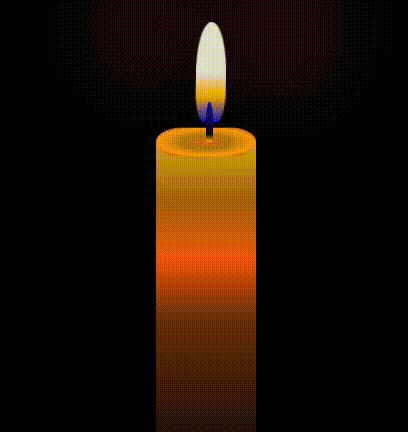# How to create Candle Animation using CSS ?

• Last Updated : 07 Oct, 2021

To create Candle animation, we take the basic approach of Pure CSS., Here we are using some animation techniques like move and rotate to create the Candle more effectively.

Approach: First we create a container class. In container class, we create another class named candle-body and in this class, we create another two class candle-stick and candle-flames. In the candle-body selector, we set the height and width of the candle body. To add the stick in the candle body, we use some CSS properties in the candle-stick selector after that we add candle flames we add some animation in the  candle-flames selector like move and rotate. We are describing some properties and selectors which we have used in the CSS file:

Attention reader! Don’t stop learning now. Get hold of all the important HTML concepts with the Web Design for Beginners | HTML  course.

• background: It is used to add background effects for elements.
• animation: The  animation is created by gradually changing from one set of CSS styles to another.
• @keyframes: The  @keyframes  rules specifies the animation code.
• gradient: It provides the smooth transitions between two or more specified colors.
• z-index: z-index sets the z-order of a positioned element.
• transform: It applies a 2D or 3D transformation to an element. It allows you to rotate, move, skew, etc., elements.

Below is the implementation of the above approach.

## HTML

 ```<``html``>`` ` `<``head``>``    ``<``title``>Candle Animation using CSS`` ` `    ````    ``<``style` `type``=``"text/css"``>``        ``.container {``            ``height: 100vh;``            ``width: 100vw;``            ``align-items: center;``            ``justify-content: center;``            ``background: rgb(26, 25, 25);``            ``animation: change-background 3s infinite linear;``        ``}`` ` `        ``.candle-body {``            ``position: absolute;``            ``width: 100px;``            ``height: 350px;``            ``background: linear-gradient(``                ``rgb(209, 158, 64),``                ``rgb(165, 96, 11),``                ``rgb(241, 85, 12),``                ``rgb(109, 47, 3) 50%,``                ``rgba(0, 0, 0, 0.6)``            ``);``            ``bottom: 1%;``            ``left: 50%``        ``}`` ` `        ``.candle-body:before {``            ``content: "";``            ``position: absolute;``        ``}`` ` `        ``.candle-body:after {``            ``content: "";``            ``position: absolute;``            ``top: -25px;``            ``height: 50px;``            ``width: 100px;``            ``border-radius: 50px;``            ``background: radial-gradient(``                ``rgb(226, 95, 34),``                ``rgb(168, 117, 23),``                ``rgb(255, 149, 18),``                ``rgb(112, 49, 3)``            ``);``             ` `            ``transform: rotateX(55deg);``            ``box-shadow: insert 2px 3px 4px ``                ``rgba(0, 0, 0, 0.2),``                ``insert -2px -3px 4px ``                ``rgba(0, 0, 0, 0.2);``        ``}`` ` `        ``.candle-stick {``            ``width: 7px;``            ``height: 40px;``            ``position: absolute;``            ``top: -40px;``            ``left: 50px;``            ``background: linear-gradient(``                ``rgb(7, 7, 204) 2%,``                ``rgb(15, 0, 0) 80%,``                ``rgb(248, 165, 11) ``                ``99%``            ``);``            ``border-radius: 50% 50% 20% 20%;``            ``z-index: 10;``        ``}`` ` `        ``.candle-flames {``            ``position: absolute;``            ``background: linear-gradient(``                ``rgb(224, 216, 216) 50%,``                ``rgb(233, 157, 17),``                ``rgb(12, 12, 226)``            ``);``            ``border-radius: 50% 50% 30% 30%;``            ``width: 30px;``            ``height: 100px;``            ``top: -120px;``            ``left: 35px;``            ``animation: move 1s linear infinite, ``                    ``rotate 2s linear infinite;``            ``box-shadow: 70px -50px 100px rgb(228, 70, 13),``                ``-70px -50px 100px rgb(180, 52, 6);``        ``}`` ` `        ``@keyframes move {``            ``0% {``                ``transform: rotateZ(1deg);``            ``}`` ` `            ``100% {``                ``transform: rotateZ(-1deg);``            ``}``        ``}`` ` `        ``@keyframes rotate {``            ``0% {``                ``top: -120px;``                ``left: 40px;``            ``}`` ` `            ``100% {``                ``top: -120px;``                ``left: 40px;``            ``}``        ``}`` ` `        ``@keyframes change-background {`` ` `            ``0%,``            ``60%,``            ``98%,``            ``100% {``                ``background: rgb(3, 52, 65);``            ``}`` ` `            ``61%,``            ``97% {``                ``background: #000;``            ``}``        ``}``    `````` ` `<``body``>``    ``<``div` `class``=``"container"``>``        ``<``div` `class``=``"candle-body"``>``            ``<``div` `class``=``"candle-stick"``>``            ``<``div` `class``=``"candle-flames"``>``        ````    `````` ` ``

Output:My Personal Notes arrow_drop_up